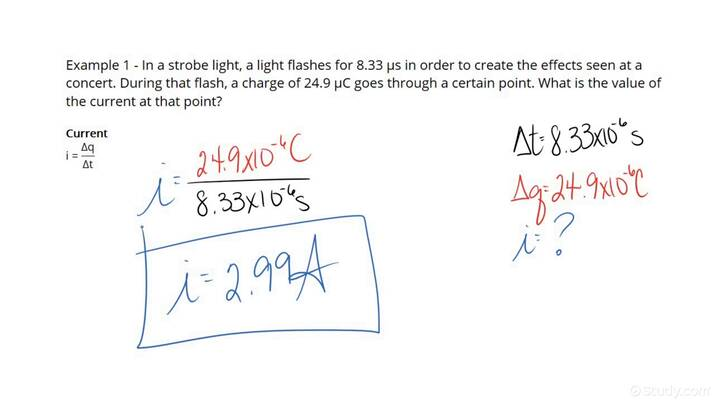# How to Find the Current in a Conductor with a Given Charge & Time IntervalAn error occurred trying to load this video.

Try refreshing the page, or contact customer support.

• 00:04 How to Find the…
• 02:33 How to Find the…
Maria El-Amin, Kirsten Wordeman
• Instructors
Maria El-Amin

Maria E. has a Bachelor’s in Physics form the University of Athens and Master’s and PhD degrees in Physics from the University of Toledo. She has 11 years of teaching experience in higher education, and 5 years of experience as a High-school principal. She has also tutored extensively.

•
Kirsten Wordeman

Kirsten has taught high school biology, chemistry, physics, and genetics/biotechnology for three years. She has a Bachelor's in Biochemistry from The University of Mount Union and a Master's in Biochemistry from The Ohio State University. She holds teaching certificates in biology and chemistry.

## How to Find the Current in a Conductor with a Given Charge & Time Interval

Step 1: Read the problem and find the values for the charge {eq}\Delta q {/eq} and the time interval {eq}\Delta t {/eq}.

Step 2: Substitute these values into the equation: $$i=\frac{\Delta q}{\Delta t}$$

Step 3: Using this equation, calculate the current {eq}i {/eq}.

## What Is the Electric Current in a Conductor?

Electric Current: It is a stream of moving charges. An example: when we connect a metal wire to a battery, the conduction electrons will move towards the positive terminal of the battery.

We define the electric current {eq}i {/eq} as the amount of charge {eq}\Delta q {/eq} that passes through a point in a conductor in a time interval {eq}\Delta t {/eq} : {eq}i=\frac{\Delta q}{\Delta t} {/eq}. The standard unit of current is {eq}1\ \mathrm{A} {/eq} (Ampere), which is equivalent to {eq}\frac{\mathrm{C}}{\mathrm{s}} {/eq} (Coulomb per second).

Electric current is drawn as an arrow that points towards the direction in which positive charges would move (if the stream consists of negative particles, the arrow points in the opposite direction of the actual flow).

Charge: It is a characteristic of matter that decides how matter behaves inside electric and magnetic fields. There are two types of charge: positive and negative. The standard unit of charge is {eq}1\ \mathrm{C} {/eq} (Coulomb).

Time interval: It is a measure of the duration of an event. The standard unit of time is {eq}1\ \mathrm{s} {/eq} (second).

We will now solve two problems (step-by-step) to enforce our understanding as to how to find the current in a conductor with a given charge & time interval.

## Examples of How to Find the Current in a Conductor with a Given Charge & Time Interval

Example 1

Find the current in a conductor with a charge of {eq}50\ \mathrm{C} {/eq} passing through a given point in 5 seconds.

Step 1: Read the problem and find the values for the charge {eq}\Delta q {/eq} and the time interval {eq}\Delta t {/eq}.

Charge: {eq}50\ \mathrm{C} {/eq}

Time interval: {eq}5\ \mathrm{s} {/eq}

Step 2: Substitute these values into the equation: \begin{align} i&=\frac{\Delta q}{\Delta t}\\ i&=\frac{(50\ \mathrm{C})}{(5\ \mathrm{s})} \end{align}

Step 3: Using this equation, calculate the current {eq}i {/eq}.

\begin{align} i&=\frac{(50\ \mathrm{C})}{(5\ \mathrm{s})}\\ i&=10\ \mathrm{A} \end{align}

Example 2

In a lightning flash, a charge of {eq}20\ \mathrm{C} {/eq} goes through a certain point in 2 milliseconds. What is the value of the current at that point?

Step 1: Read the problem and find the values for the charge {eq}\Delta q {/eq} and the time interval {eq}\Delta t {/eq}.

Charge: {eq}20\ \mathrm{C} {/eq}

Time interval: {eq}2\ \mathrm{ms} {/eq}. To convert to seconds we use the relationship: {eq}1\ \mathrm{ms}=0.001\ \mathrm{s} {/eq}. Our time interval is {eq}0.002\ \mathrm{s} {/eq}.

Step 2: Substitute these values into the equation: \begin{align} i&=\frac{q}{\Delta t}\\ i&=\frac{(20\ \mathrm{C})}{(0.002\ \mathrm{s})} \end{align}

Step 3: Using this equation, calculate the current {eq}i {/eq}.

\begin{align} i&=\frac{(20\ \mathrm{C})}{(0.002\ \mathrm{s})}\\ i&=10,000\ \mathrm{A} \end{align}